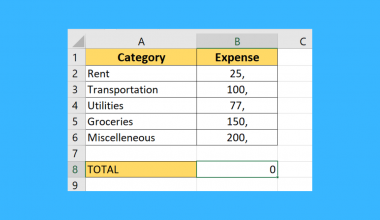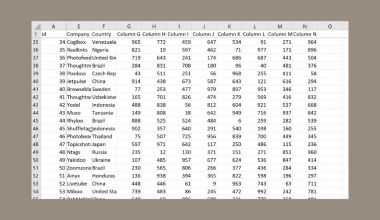# How to Trace Precedents and Dependents in Excel

This guide will explain how to trace precedents and dependents in Excel.

Understanding the precedents and dependents of a cell can help you understand the relations between cells in your spreadsheet.

When working with sheets with dozens of formulas, you may need help understanding how each cell relates to one another.

A single formula can use multiple cells as arguments. The value of a single cell may also appear in the formulas of other cells. How can we determine what cells in our sheet use a particular cell’s value?

Excel provides two features we can use to show cell relations: Trace Precedents and Trace Dependents.

The Trace Precedents command shows all cell references in the current cell. For example, if cell B2 contains the formula `=C5`, then cell C5 is considered a precedent to cell B2.

The Trace Dependents command, on the other hand, shows formulas that use the value of a particular cell. In our earlier example, cell B2 is considered a dependent cell to C5 since it uses a cell reference to C5 in its formula.

We can use both these commands to troubleshoot errors in our cell. For example, if a formula returns a `#DIV/0` error, we can use the Trace Precedents command to see if any of the cell references used in the formula is causing the error.

Similarly, we can use the Trace Dependents command to look for cells that are affected by the error.

Now that we have a grasp on how precedent and dependent cells could help us understand our sheet, let’s see how it works on an actual spreadsheet.

## A Real Example of Tracing Precedents and Dependents in Excel

The following section provides several examples of how to trace precedents and dependents in Microsoft Excel.

In the spreadsheet below, we have several cells that contain formulas. If you are unfamiliar with what each cell does, it may be difficult to understand how each cell relates to the other. We can use an Excel command to determine what other cells in your spreadsheet relate to the current cell.For example, we can use the Trace Dependents command while cell I3 is selected to determine which other cells use the population mean value. Similarly, we can use the Trace Precedents command to know which values cell I3 uses to calculate the population mean.If you’ve encountered an error in your spreadsheet, you can use these commands to troubleshoot each formula.

In the example below, we have a #NUM! error in cell I6. We can use the Trace Precedents command to determine that cells I4 and I5 were used to obtain the result.Using the Trace Precedents command again, we’ve discovered that the formula calculating the population size uses an incorrect cell reference.Do you want to take a closer look at our examples? You can make your own copy of the spreadsheet above using the link attached below.

If you’re ready to try out using these commands yourself, head over to the next section to read our detailed guide on how to use them!

## How to Trace Precedents and Dependents in Excel

This section will guide you through each step needed to trace precedents and dependents of specific cells in your spreadsheet. You’ll learn how we can use these commands to solve errors and make sense of complex worksheets.

Follow these steps to start using the Trace Precedents and Trace Dependents commands:

1. First, let’s look at how to use the Trace Precedents command. We can use this feature to determine which cells are used to calculate a particular formula.

Select the cell you want to know the precedents of. In this example, we want to illustrate where the value for the population mean in cell I3 comes from.2. In the Formulas tab, click on the ‘Trace Precedents’ option.3. Excel will highlight the cell or cell ranges that are part of your current formula. The arrow’s direction shows where the data flows. In the example below, the cell range that covers our test scores calculates the population mean.4. Next, we’ll try to use the Trace Dependents command. Select the cell you want to apply this command to. In this example, we want to know what cells use the confidence value in cell I6.5. Similar to the Trace Precedents command, the Trace Dependents feature can be found in the Formulas tab.6. Excel will use a blue arrow to indicate which cells use the value found in the currently selected cell. In this example, we can see that the value in cell I6 is referenced in cells I8 and I9.7. To remove the blue arrows generated by these commands, click on the Remove Arrows option in the Formulas tab.8. You may also expand the dropdown menu to select additional options. We can use the other options to remove only precedent or dependent arrows.This step-by-step guide is a quick introduction to using the Trace Precedents and Trace Dependents command in Microsoft Excel.

Overall, these commands are a quick way to understand the relationship between various formulas in your spreadsheet. The arrows also help illustrate how the data flows in your spreadsheet.

This function is just one example of the many Excel commands you can apply to your spreadsheets. Our website offers hundreds of other functions and methods to help you get more out of Microsoft Excel.

With so many other Excel functions available, you can find one that is appropriate for your use case.

Subscribe to our newsletter, and you’ll get exclusive access to the latest spreadsheet guides and tutorials from us.### Get emails from us about Excel.

Our goal this year is to create lots of rich, bite-sized tutorials for Excel users like you. If you liked this one, you'd love what we are working on! Readers receive ✨ early access ✨ to new content.

##### You May Also Like## How to Ignore Blanks When Calculating the Weighted Average in Excel

Learning how to ignore blanks when calculating the weighted average in Microsoft Excel is useful when you want…## How to Calculate Cost of Funds in Excel

This guide will discuss how to calculate the cost of funds in Excel. The cost of funds is…## How to Lookup Partial String Match in Excel

The VLOOKUP function is useful when you need to lookup a partial string match in Excel. We can…## How to Reduce Decimals in Excel

This guide will explain several methods to reduce decimals in Excel. Excel comes with several formatting options and…